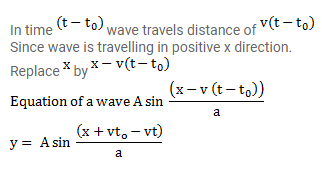# A wave propagates on a string with a speed v.`
Question:

A wave propagates on a string with a speed $v$. The shape of the string at $t=t_{0}$ is given by $g\left(x, t_{0}\right)=A$ sin $(x / a)$. Write the wave equation for a general time t.

Solution: